Smartick is an advanced online program that teaches kids math and coding in only 15 min. a day

Jul26

# Learn How to Subtract Fractions

In today’s post, we are going to look at how to subtract fractions; but in order subtract them, first you’ll have to know what the denominator and numerator mean in a fraction:

The numerator is the number that is written in the top part of the fraction and the denominator is the number that is written in the bottom part of the fraction.Now, you just need to keep in mind that fractions need the have the same denominator in order to subtract them. Once the fractions have the same denominator, we just need to subtract the numerators.

What do we do if the fractions have different denominators? In this case, we need to find the same denominator by calculating their least common multiple (l.c.m.)

Let’s look at an example: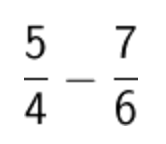The denominators are different, 4 and 6, so we need to solve for their least common multiple.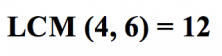The two new fractions will have the number 12 as their denominator.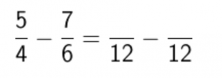In order to calculate the numerator for each new fraction, we divide the new denominator (the l.c.m. that we found before) by the old denominator and we’ll multiply the answer by the old numerator.

The first fraction: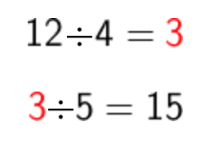The second fraction: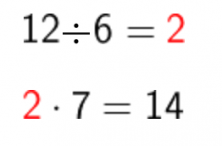Now subtracting the fractions gives us: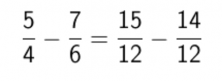The two fractions have the same denominators now, so we can subtract: subtract the numerators and leave the denominator as is: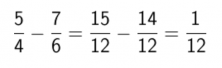The final answer that we get from subtracting the fractions is one-twelfth.

If you liked this post, share it with your friends so they can learn how to subtract fractions, too!